Courses

# Test: Integrals Of Trigonometric Identities

## 5 Questions MCQ Test Mathematics For JEE | Test: Integrals Of Trigonometric Identities

Description
This mock test of Test: Integrals Of Trigonometric Identities for JEE helps you for every JEE entrance exam. This contains 5 Multiple Choice Questions for JEE Test: Integrals Of Trigonometric Identities (mcq) to study with solutions a complete question bank. The solved questions answers in this Test: Integrals Of Trigonometric Identities quiz give you a good mix of easy questions and tough questions. JEE students definitely take this Test: Integrals Of Trigonometric Identities exercise for a better result in the exam. You can find other Test: Integrals Of Trigonometric Identities extra questions, long questions & short questions for JEE on EduRev as well by searching above.
QUESTION: 1

### The integral of tan4x is:

Solution:

Begin by rewriting  ∫tan4xdx as ∫tan2xtan2xdx.

Now we can apply the Pythagorean Identity,  tan2x+1=sec2x,                                         or tan2x=sec2x−1

∫tan2x tan2x dx = ∫(sec2x−1)tan2xdx

Distributing the tan2x:
= ∫sec2xtan2x − tan2xdx
Applying the sum rule:
= ∫sec2xtan2xdx − ∫tan2xdx

We'll evaluate these integrals one by one.

First Integral
This one is solved using a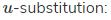Let u = tanx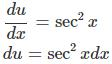Applying the substitution,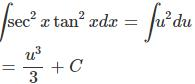Because u = tanx,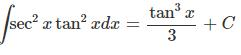Second Integral
Since we don't really know what  ∫tan2xdx is by just looking at it, try applying the tan2x = sec2x−1

identity again:

∫tan2xdx = ∫(sec2x−1)dx

Using the sum rule, the integral boils down to:
∫sec2xdx − ∫1dx

The first of these,  ∫sec2xdx, is just tanx + C.

The second one, the so-called "perfect integral", is simply x+C.

Putting it all together, we can say:
∫tan2xdx = tanx + C − x + C

And because C+C is just another arbitrary constant, we can combine it into a general constant C:
∫tan2xdx = tanx − x + C

Combining the two results, we have:
∫tan4xdx=∫sec2xtan2xdx−∫tan2xdx

=(tan3x/3 + C) − (tanx − x + C)

=tan3x/3 − tanx + x + C

Again, because C+C is a constant, we can join them into one C.

QUESTION: 2

### Integrate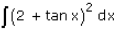Solution:

∫(2+tan x)2dx
= ∫(4 + tan2 x + 4tan x)dx
= ∫4 dx + ∫tan2 x dx + 4∫tan x dx
= 4x + ∫(sec2 x - 1)dx + 4(log|sec x|)
= 4x + tanx - x + 4(log|sec x|)
3x + tanx + 4(log|sec x|) + c

QUESTION: 3

### Evaluate: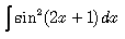Solution:

∫sin2(2x+1) dx
Put t = 2x+1
dt = 2dx
dx= dt/2
= 1/2∫sin2 t dt
=1/2∫(1-cos2t)/2 dt
= 1/4∫(1-cos2t) dt
= ¼[(t - (sin2t)/2]dt
= t/4 - sin2t/8 + c
= (2x+1)/4 - ⅛(sin(4x+2)) + c
= x/2 - 1/8sin(4x+2) + ¼ + c
As ¼ is also a constant, so eq is = x/2 - 1/8sin(4x+2) + c

QUESTION: 4

The value of  bb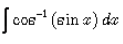Solution:

Let cos−1(sinx)=θ
⇒ sinx=cosθ
⇒ sinx=sin(π/2−θ)
⇒ x = π/2−θ
⇒ θ = π/2−x
∴ ∫cos−1(sinx)dx=∫(π/2−x)dx
= ∫π/2dx−∫xdx
= πx/2 - x2/2 + c, where b is a constant of integration.

QUESTION: 5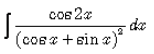Solution:

I = ∫cos2x/(sinx+cosx)2dx
⇒I = ∫cos2x−sin2x(sinx+cosx)2dx
⇒I = ∫[(cosx+sinx)(cosx−sinx)]/(sinx+cosx)2dx
⇒I = ∫(cosx−sinx)/(sinx+cosx)dx
Let sinx+cosx = t
(cosx−sinx)dx = dt
Then, I = ∫dt/t
I = log|t|+c
I = log|sinx + cosx| + c